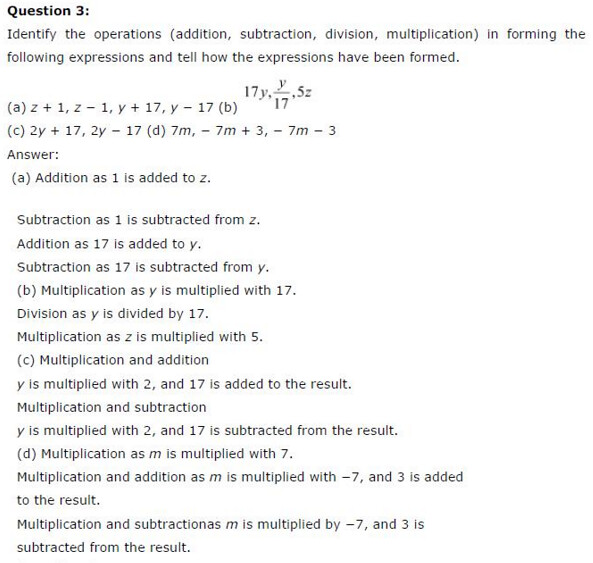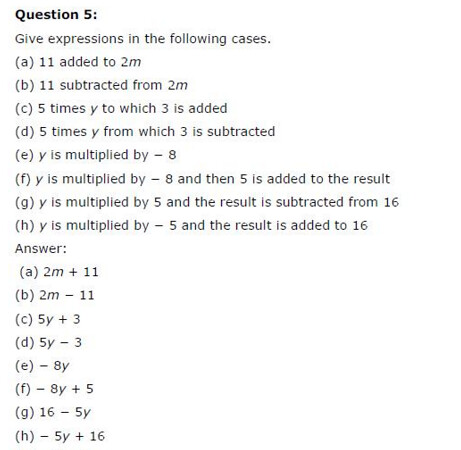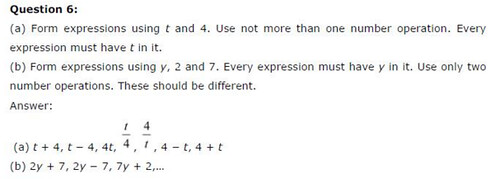# NCERT Solutions For Class 6 Maths Algebra Exercise 11.3

NCERT Solutions For Class 6 Maths Algebra Exercise 11.3

### NCERT Solutions For Class 6 Maths Algebra Exercise 11.3

NCERT Solutions For Class 6 Maths Chapter 11 Algebra Ex 11.3

Exercise 11.3

Ex 11.3 Class 6 Maths Question 1.
Make up as many expressions with numbers (no variables) as you can from three numbers 5, 7 and 8. Every number should be used not more than once. Use only addition, subtractions and multiplication.
Solution:
Given numbers are 5, 7 and 8.
Expressions are:
(i) 8 + (5 + 7)
(ii) 5 + (8 – 7)
(iii) 8 + (5 x 7)
(iv) 7 – (8 – 5)
(v) 7 x (8 + 5)
(vi) 5 x (8 + 7)
(vii) 8 x (5 + 7)
(viii) 7 + (8 – 5)
(ix) (5 x 7) – 8
(x) 7 + (8 x 5)

Ex 11.3 Class 6 Maths Question 2.
Which out of the following are expressions with numbers only?
(a) y + 3
(b) (7 x 20) – 8z
(c) 5(21 – 7) + 7 x 2
(d) 5
(e) 3x
(f) 5 – 5n
(g) (7 x 20) – (5 x 10) – 45 +p
Solution:
(a) y + 3. This expression has variable ‘y’.
(b) (7 x 20) – 8z. This expression has a variable ‘z’.
(c) 5(21 -7) + 7 x 2. This expression has no variable. So it is with numbers only.
(d) 5. This expression is with numbers only.
(e) 3x. This expression has a variable ‘x’.
(f) 5 – 5n. This expression has a variable ‘n’.
(g) (7 x 20) – (5 x 10) – 45 + p. This expression has a variable ‘p’.

Ex 11.3 Class 6 Maths Question 3.
Identify the operations (addition, subtraction, division and multiplication) in forming the following
expressions and tell how the expressions have been formed.
(a) z + 1, z – 1,y + 17, y – 17
(b) 17y,y/17 , 5z
(c) 2y + 17, 2y – 17
(d) 7m, -7m + 3, -7m – 3
Solution:

 Expressions Operations used Formation of expression (a) (i) z + 1 Addition z is increased by 1 (ii) z – 1 Subtraction z is decreased by 1 (iii) y +17 Addition y is increased by 17 (iv) y -17 Subtraction y is decreased by 17 (b) (i) 17y Multiplication y is multiplied by 17 (ii) y/17 Division y is Divided by 17 (iii) 5z Multiplication z is Multiplied by 5 (c) (i) 2y + 17 Multiplication and addition y is multiplied by 2 and then 17 is added. (ii) 2y -17 Multiplication and subtraction Twice of y is decreased by 17 (d) (i) 7 m Multiplication m is multiplied by 7 (ii)- -7m + 3 Multiplication and addition m is multiplied by -7 and then increased by 3 (iii) -7m – 3 Multiplication and subtraction M is multiplied by -7 and then decreased by 3

Ex 11.3 Class 6 Maths Question 4.
Give expressions for the follow
(b) 7 subtracted from p
(c) p multiplied by 7
(d) p divided by 7
(e) 7 subtracted from -m
(j) -p multiplied by 5
(g) -p divided by 5
(h) p multiplied by -5
Solution:Ex 11.3 Class 6 Maths Question 5.
Give expressions in the following cases:
(b) 11 subtracted from 2m
(c) 5 times y to which 3 is added
(d) 5 times y from which 3 is subtracted
(e) y is multiplied by -8
(f) y is multiplied by -8 and then 5 is added to the result
(g) y is multiplied by 5 and the result is subtracted from 16
(h) y is multiplied by -5 and the result is added to 16.
Solution:
(a) 2m + 11
(b) 2m – 11
(e) 5y + 3
(d) 5y – 3
(e) -8y
(f) -8y+5
(g) 16 – 5y
(h) -5y + 16

Ex 11.3 Class 6 Maths Question 6.
(a) Form expressions using t and 4. Use not more than one number operation. Every expression must have t in it.
(b) Form expressions using y, 2 and 7. Every expression must have y in it. Use only two number operations. These should, be different.
Solution:
(a) The possible expressions are:
(i) t + 4
(ii) t – 4
(iii) 4t
(iv) t/4
(v) 4 + t
(vi) 4 + t,etc.

(b) The possible expressions are:## SabDekho

The Complete Educational Website